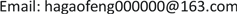1. 引言

P = ω 1 X 1 + ω 2 X 2 + ⋯ + ω p X p (1.1)

I ( X 1 , ⋯ , X p ) − I P ( ω 1 , ⋯ , ω p ) < I ( X 1 , ⋯ , X p ) − I P ( ω ′ 1 , ⋯ , ω ′ p )

I P ( ω 1 0 , ⋯ , ω p 0 ) = max { ω 1 , ⋯ , ω p } { I P ( ω 1 , ⋯ , ω p ) }

2. 主要结果2.1. 最优标准评价量

1) 该二级指标的最优标准评价量为

P = e 1 T X

2) 最优标准评价量的信息损失比为

1 − λ 1 ∑ i = 1 p λ i

P = ω 1 X 1 + ω 2 X 2 + ⋯ + ω p X p = ω T X ，其中 ∑ i = 1 p ω i 2 = 1 ，

max V a r ( P ) = max ‖ ω ‖ = 1 ( ω T Σ ω )

∑ i = 2 p λ i ∑ i = 1 p λ i = 1 − λ 1 ∑ i = 1 p λ i

2.2. 上市公司投资价值评价的一种新方法

X = μ + Q F + ε (2.1)

Φ = Q Q T + Ψ (2.2)

3. 实证分析

QFII held part of the awkwardness in 201

f 1f 2f 3f 4f 1f 2f 3f 4
X 10.34340.09930.7329−0.1865X 80.97810.0178−0.0341−0.1970
X 2−0.2062−0.0018−0.03240.7278X 90.95760.11400.0307−0.2548
X 30.0044−0.16520.56330.1446X 100.65160.73970.16780.0078
X 40.19280.16120.13400.3830X 110.13790.8950−0.12330.1559
X 5−0.17110.38840.87560.0687X 12−0.2667-0.0942−0.14600.4863
X 6−0.04860.97180.17630.0525X 13−0.15410.22960.18380.4631
X 70.99070.04700.0832−0.0616

Common factors interpretatio

z i j = x i j − x ¯ j s j ,   i = 1 , ⋯ , 20 ; j = 1 , ⋯ , 13 ，

P i 1 = 0.5757 z i 7 + 0.5789 z i 8 + 0.5774 z i 9 ,   i = 1 , ⋯ , 20

P i j − min 1 ≤ i ≤ 20 ( P i j ) max 1 ≤ i ≤ 20 ( P i j ) − min 1 ≤ i ≤ 20 ( P i j ) ,     i = 1 , ⋯ , 20 , j = 1 , 2 , 3 , 4 ，

Evaluation value

P 0 = 0.3062 P 1 + 0.2615 P 2 + 0.2137 P 3 + 0.2186 P 4

4. 结论

1) 各个二级指标的信息损失比可能相差较大，其中盈利能力的信息损失比仅仅为1.4%，说明盈利能力的评价结果是很准确的，而成长能力的信息损失比高达47.2%，所以它的评价结果是不够准确的，并且受它的影响，导致了综合最优标准评价量出现负权重的不合理现象。

2) 我们的评价方法不仅能够得到最优标准评价量，而且还能够根据信息损失比，分析评价结果的准确性，信息损失比越小，则评价结果越准确。

3) 与文献  中的评价结果相比较，在盈利能力指标上，两者在位次上的一致性达到90%，而在其余的三个指标上，两者的一致性不高。说明当信息损失比很小时，改进的熵权法的评价效果能接近于最优标准评价量。